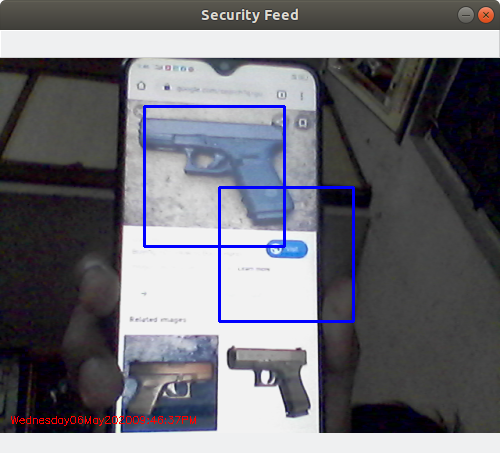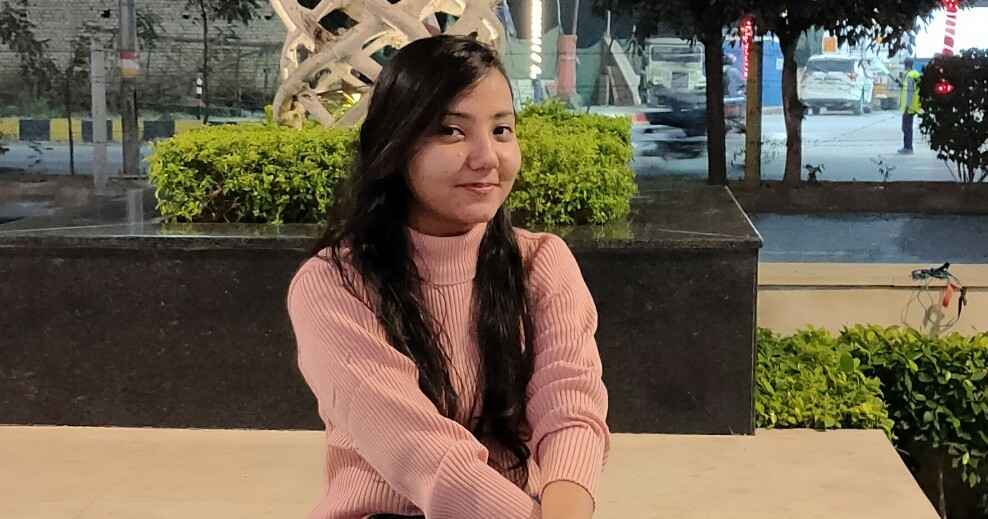# Gun Detection using Python-OpenCV

Prerequisites: Python OpenCV

Gun Detection using Object Detection is a helpful tool to have in your repository. It forms the backbone of many fantastic industrial applications. OpenCV(Open Source Computer Vision Library) is a highly optimized library with focus on Real-Time Applications.

Approach:

1) Creation of Haarcascade file of Guns: Refer to Creation of own haarcascade

From here, you will learn about how to create your own Haarcascade file. With your single positive image, you can use the opencv_createsamples command to actually create a bunch of positive examples, using your negative images. Your positive image will be superimposed on these negatives, and it will be angled and all sorts of things. It actually can work pretty well, especially if you are really just looking for one specific object. If you are looking to identify all guns, however, you will want to have thousands of unique images of guns, rather than using the opencv_createsamples to generate samples for you. We’ll keep it simple and just use one positive image, and then create a bunch of samples with our negatives.

2) Detection of Guns using OpenCV

 `import` `numpy as np ` `import` `cv2 ` `import` `imutils ` `import` `datetime ` ` `  `  `  `gun_cascade ``=` `cv2.CascadeClassifier(``'cascade.xml'``) ` `camera ``=` `cv2.VideoCapture(``0``) ` `  `  `firstFrame ``=` `None` `gun_exist ``=` `False` `  `  `while` `True``: ` `     `  `    ``ret, frame ``=` `camera.read() ` `  `  `    ``frame ``=` `imutils.resize(frame, width ``=` `500``) ` `    ``gray ``=` `cv2.cvtColor(frame, cv2.COLOR_BGR2GRAY) ` `      `  `    ``gun ``=` `gun_cascade.detectMultiScale(gray, ` `                                       ``1.3``, ``5``, ` `                                       ``minSize ``=` `(``100``, ``100``)) ` `      `  `    ``if` `len``(gun) > ``0``: ` `        ``gun_exist ``=` `True` `          `  `    ``for` `(x, y, w, h) ``in` `gun: ` `         `  `        ``frame ``=` `cv2.rectangle(frame, ` `                              ``(x, y), ` `                              ``(x ``+` `w, y ``+` `h), ` `                              ``(``255``, ``0``, ``0``), ``2``) ` `        ``roi_gray ``=` `gray[y:y ``+` `h, x:x ``+` `w] ` `        ``roi_color ``=` `frame[y:y ``+` `h, x:x ``+` `w]     ` `  `  `    ``if` `firstFrame ``is` `None``: ` `        ``firstFrame ``=` `gray ` `        ``continue` `  `  `    ``# print(datetime.date(2019)) ` `    ``# draw the text and timestamp on the frame ` `    ``cv2.putText(frame, datetime.datetime.now().strftime(``"% A % d % B % Y % I:% M:% S % p"``), ` `                ``(``10``, frame.shape[``0``] ``-` `10``), ` `                ``cv2.FONT_HERSHEY_SIMPLEX, ` `                ``0.35``, (``0``, ``0``, ``255``), ``1``) ` `  `  `    ``cv2.imshow(``"Security Feed"``, frame) ` `    ``key ``=` `cv2.waitKey(``1``) & ``0xFF` `     `  `    ``if` `key ``=``=` `ord``(``'q'``): ` `        ``break` ` `  `        ``if` `gun_exist: ` `    ``print``(``"guns detected"``) ` `else``: ` `    ``print``(``"guns NOT detected"``) ` ` `  `camera.release() ` `cv2.destroyAllWindows() `

Output:OpenCV comes with a trainer as well as a detector. If you want to train your own classifier for any object like car, planes, etc. you can use OpenCV to create one.

Here we deal with the detection of Gun. First we need to load the required XML classifiers. Then load our input image (or video) in grayscale mode. Now we find the guns in the image. If guns are found, it returns the positions of detected guns as `Rect(x, y, w, h)`. Once we get these locations, we can create a ROI(Region of Interest) for the gun.

My Personal Notes arrow_drop_upCheck out this Author's contributed articles.

If you like GeeksforGeeks and would like to contribute, you can also write an article using contribute.geeksforgeeks.org or mail your article to contribute@geeksforgeeks.org. See your article appearing on the GeeksforGeeks main page and help other Geeks.

Please Improve this article if you find anything incorrect by clicking on the "Improve Article" button below.

Article Tags :

2

Please write to us at contribute@geeksforgeeks.org to report any issue with the above content.